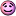## Recommended Posts

OK, please be gentle, i'm really not good at physics.

As i understand it, there's 3 forces: strong, electroweak, and gravity.

Other forces, such as magentism, can be described in terms of electroweak (?); i.e., it's not a unique force, rather a different incarnation of electroweak, or something like that. heat is not a unique force, it's just an incarnation of movement (i.e., the amount of movement of the molecules).

But, i can't think of what motion is. it's clearly an energy, as it can be converted to/fro the other energies, so if it weren't an energy it'd break the first law; but, i can't see how it could be an arrangement of strong, electroweak and/or gravity.

I'll admit i'm not 100% sure what strong and electroweak areSo, yeah, what's motion wrt energy please?

##### Share on other sites

some say motion is an illusion... however a good book to start with is motion mountain a physics textbook it is well written for the beginner.. You can download it in pdf format and free as well. They discuss what is motion is depth

##### Share on other sites

OK, please be gentle, i'm really not good at physics.

As i understand it, there's 3 forces: strong, electroweak, and gravity.

Other forces, such as magentism, can be described in terms of electroweak (?); i.e., it's not a unique force, rather a different incarnation of electroweak, or something like that. heat is not a unique force, it's just an incarnation of movement (i.e., the amount of movement of the molecules).

But, i can't think of what motion is. it's clearly an energy, as it can be converted to/fro the other energies, so if it weren't an energy it'd break the first law; but, i can't see how it could be an arrangement of strong, electroweak and/or gravity.

I'll admit i'm not 100% sure what strong and electroweak areSo, yeah, what's motion wrt energy please?

All three of these forces can effect motion of an object, a displacement over time in a reference frame.

##### Share on other sites

but how do they affect motion?

(if anyone's familiar with my spelling, affect is spelt correctly there: how do they actually cause motion? what is motion?)

##### Share on other sites

Well, simply force is equal to the time derivative of momentum.

$\mathbf{F}=\frac{d(m\mathbf{v})}{dt}$

F = force

m = mass of object

v = velocity

(force and velocity are bolded because they are vectors)

very often, the mass of an object is a constant (only things like rockets which expel mass would have a changing m), so that can be taken out of the derivative

$\mathbf{F}=m\frac{d\mathbf{v}}{dt}$

velocity is the time derivative of position

$\mathbf{v} = \frac{d\mathbf{x}}{dt}$

So, velocity is the change in position with respect to time (that is, motion), and a force is the change of velocity with respect to time, and that force can some from any from your list. That's how a force and motion are related mathematically in physics.

##### Share on other sites

Motion is a change in the relative position of an object. The rate of this change with respect to time is velocity. The rate of change of velocity with respect to time is acceleration.

##### Share on other sites

(To note there are normally considered to be 4 fundemental forces, Electromagnetic, weak, strong and graivty. Electromagenetic was unified by Maxwell and is unified for energies we experience. EM and weak are unified for higher energies than are normally experienced (100GeV), there is strong evidence that a Grand Unification Theory, for energies greater than 1014GeV will be able to be created (there are several such field theories atm) unifying EM, Weak and Strong. And then of course there is the idea of a theory of everything, that wouid unify all 4, that would probably be for very very very high energies and it's anyones guess which one of the many many ideas will turn out to be true, if any of them)....

As for "what is motion" other than a change in relative position as commented above it's a very difficult thing to describe in words...

##### Share on other sites

You can describe motion without reference to forces at all, though they are related by the equations Bignose has given. But remove the mass from the relationships and you have how acceleration, velocity and displacement are connected.

## Create an account

Register a new account